# Glossary of Terms

## ASTM

American Society for Testing and Materials.

## Blending

The thorough intermingling of powders having the same nominal composition. For example, blending two different lots of the same raw materials to smooth out any minor variations.

## Density

Density is the ratio of mass, to volume.

## Differential Scanning Calorimetry (DSC)

Differential Scanning Calorimetry is a technique in which the heat flow (thermal power) of the sample is measured as a function of time or temperature when the temperature of this sample is scanned, in a controlled atmosphere. In practice what is measured is the difference in heat flow between a crucible containing the sample and a reference crucible (the latter is generally empty, but may also contain a material which is thermally inert in the temperature range being studied).

## Diffusivity

Thermal diffusivity is the measure of the way heat flows through a material to the other side. It is also often expressed as the rate of change of temperature in a transient heat transfer process. The higher the thermal diffusivity of a material, the higher the rate of temperature propagation.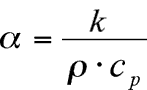Where: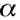= Thermal Diffusivity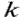= Thermal Conductivity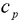= Specific Heat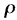= Density

According to this relation, thermal diffusivity will affect any conductive transient heat transfer process within the sample medium. Thermal diffusivity has the dimension length2 / time and is expressed in the units’ mm2/s.

## Dispersion

Dispersion is the act or process of scattering or dispersing, or the state of being scattered from one component into another.

## Drying

To remove the moisture from; make dry.

## Effusivity

Effusivity is a heat transfer property present in all materials in all formats – solid, liquid, pastes, powder and gas. Effusivity is the property that dictates the interfacial temperature when two semi-infinite objects at different temperature touch. Effusivity combines thermal conductivity, density and heat capacity into one value.

and

### Effusivity =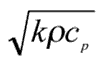Where:= Thermal Conductivity (W/m•K)= Density (kg/m3)= Heat Capacity (l/kg•K)

## Guarded Hot Plate

Guarded hot plate is a steady state technique that involves placing a solid sample of fixed dimension between two temperature controlled plates. One plate is heated while the other is cooled and temperatures of the plates are monitored until they are constant. The steady state temperatures, the thickness of the sample and the heat input to the hot plate are used to calculate the thermal conductivity.

## Specific Heat Capacity

Specific heat capacity is by definition the quantity of heat required to raise the temperature of a unit of mass of a substance by a unit change in temperature. That is: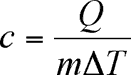OR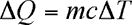Where: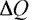= change in heat in Joules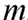= the mass in kg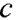= specific heat capacity in J/(kg-°C)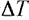= change in temperature in °C or K

The derived units for c, the specific heat capacity, is (J/kg-°C). The specific heat capacity of a substance depends on its molecular structure and on its phase.

## Homogeneity

Homogeneity is the quality of being uniform throughout in composition or structure.

## Hot Wire

Hot wire is a transient technique that involves inserting an electrically heated wire into a material. This intrusive method is possible for foams and fluids and melted plastics. The heat flows out radially from the wire into the sample and the temperature of the wire is measured. The plot of the wire temperature versus the logarithm of time is used to calculate thermal conductivity.

## Interfacial

Relating to or situated at an interface; "an interfacial layer"; "interfacial tension is the surface tension at the interface between two liquids".

## Laser Flash Diffusivity

Laser flash diffusivity is a transient method that involves applying a short pulse of heat to the front face of a specimen using a laser flash, and measuring the temperature change of the rear face with an infrared (IR) detector. The resulting temperature rise of the other face of the test specimen is monitored as a function of time and used, together with the sample thickness, to determine the thermal diffusivity. This can be combined with density and heat capacity data to calculate thermal conductivity.

## Mixing

The thorough intermingling of two or more materials.

## Modified Hot Wire

Modified hot wire is a transient heat reflectance technique similar to hot wire. The modification is that the heating element is supported on a backing, which provides a rectangular one dimensional heat flow. This eliminates the intrusive nature and allows for solids to be tested without being melted. The plot of the wire temperature versus the square root of time is used to calculate thermal conductivity, provided that density and heat capacity are known.

## Modulated Differential Scanning Calorimetry (DSC)

Modulated or dynamic differential scanning calorimetry (M or DDSC) is a technique that involves conducting two DSC measurements on samples of differing thickness. The difference in apparent heat capacity is the result of the lag in the heat penetrating through the sample during testing. The DSC results and the thicknesses of the two samples are used to calculate thermal conductivity.

## Non-destructive

Of, relating to, or being a process that does not result in damage to the material under investigation or testing.

## R-Value

R-value is a measure of the thermal resistance of a material. Thermal resistance is an index of a materials' resistance to the flow of heat. It is the reciprocal of the thermal conductivity.

## Reflectance

Reflectance is the fraction of radiant energy that is reflected from a surface.

## Thermal Conductivity

Thermal conductivity is a physical property of a material that characterizes the ability of that substance to transfer heat. The value of thermal conductivity determines the quantity of heat passing per unit of time per unit area at a temperature drop of 1-degree C per unit length. In the limit of infinitesimal thickness and difference in temperature, the fundamental law of heat conduction is: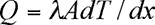Where: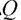is a measure of the heat flow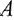is the cross sectional area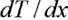is the temperature / thickness gradientis defined as the thermal conductivity

Thermal conductivity differs with each substance and may depend on the structure, density, humidity, pressure and temperature. Materials having a large thermal conductivity value are good conductors of heat; one with a small thermal conductivity value is a poor heat conductor i.e. good insulator. Hence, knowledge of the thermal conductivity value (units W/m•K) allows for quantitative comparisons to be made between the thermal insulation efficiencies of different materials.

## Thermal Transmission Properties

Thermal transmission properties are those properties of a material or system that define the ability of the material or system to transfer heat. Properties, such as thermal resistance, thermal conductance, thermal condutivity, and thermal resistivity would be included.

## Transient

Transient plane source is a type of modified hot wire. It is a transient reflectance technique for solids and liquids in which the sample surrounds a heating element configured as a planar circle. With the constant current heat input to the sample, a 3 dimensional heat flow results and the temperature of the interface is monitored and plotted against a time function. The results are thermal conductivity and diffusivity – both independent of each other, without requiring sample geometry information, density or heat capacity.

## Wetting

To fill or moisten with water or other liquid.

Visit Wikipedia, the free encyclopedia for terms related to Thermal Conductivity.